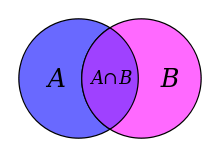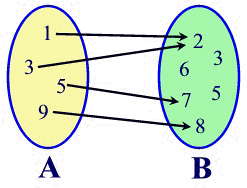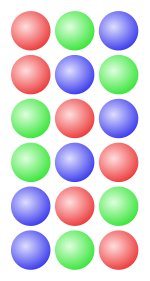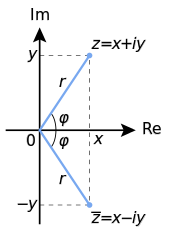×#### Thank you for registering.

One of our academic counsellors will contact you within 1 working day.

Click to Chat

1800-1023-196

+91-120-4616500

CART 0

• 0

MY CART (5)

Use Coupon: CART20 and get 20% off on all online Study Material

ITEM
DETAILS
MRP
DISCOUNT
FINAL PRICE
Total Price: Rs.

There are no items in this cart.
Continue Shopping```AlgebraAlgebra is one of the building blocks of Mathematics in IIT JEE examination. In fact, in the preparation of JEE this is the starting point. Algebra is a very scoring and an easy portion in the Mathematics syllabus of JEE. Though Algebra begins with Sets and Relations but we seldom get any direct question from this portion. Functions can be said to be a prerequisite to Calculus and hence it is critical in IIT JEE preparation. Sequence and series is another section which is mixed with other concepts and then asked in the examination. Quadratic equations fetch direct questions too and are also easy to grasp. Binomial Theorem is also a marks fetching topic as the questions on this topic are quite easy. Permutations and Combinations along with Probability is the most important section in Algebra. IIT JEE exam fetches a lot of questions on them. Those who get good IIT JEE rank always do well in this section. Complex Numbers are also important as this fetches question in the IIT JEE exam almost every year. Matrices and Determinant mostly give direct question and there are no twist and turns in the questions based on them.

We cover some of these contents here in brief as they have been discussed in detail in the coming sections:

Sets: A set is a well-defined collection of distinct objects. The different members of a set are called the elements of the set. Moreover all the elements are unique.

Example: {1, 2, 3, 6} represents a set of numbers less than 10. Note that there is no repetition of elements in a set.Relations and Functions: A relation is a set of ordered pairs. A function is a relation for which each value from the set the first components of the ordered pairs is associated with exactly one value from the set of second components of the ordered pair.

The first elements in the ordered pairs i.e. the x values form the domain while the second elements i.e. the y-values form the range. But, only the elements used by the relation are counted in the range.

The figure given below describes a mapping which shows a relation from set A to set B. As is clear from the figure, the relation consists of ordered pairs (1, 2), (3, 2), (5, 7), and (9, 8). The domain is the set {1, 3, 5, 9} and the range which is the dependent variable is the set {2, 7, 8}. Note that 3, 5, 6 are not a part of the range as they are not associated to any member of the first set.Example: f(x) = x/2 is a function because for every value of x we get another value x/2, so f(2) = 1

f (3) = 3/2

f (-6) = -3.

Functions:

Quadratic Equations: An equation of the form ax2 + bx +c = 0

where x represents the variable and a,b,c are constants with a not equal to zero.

If a = 0, the equation becomes linear and is no more a quadratic equation. An equation must have a second degree term in order to be a quadratic equation.

We give the formula of solving the quadratic equation:

The general form of quadratic equation is ax2+bx+ c

Dividing by a, we obtain

x2 + b x/a = -c /a

(x+ b/2a)2 = -c/a + b2 / 4a2 = (b2-4ac) / 4a2

x+ b/2a = ±√b2-4ac / 2a

Solving this for x we get

x = (-b±√b2-4ac) / 2a

The above equation is called the quadratic formula.

To get an idea about the types of questions asked, look into the Papers of Previous Years.

Binomial Theorem: The binomial theorem is used for expanding binomial expressions (a+b) raised to any given power without direct multiplication. Mathematically a binomial theorem can be defined as the theorem that gives the expansion of any binomial raised to a positive integral power say n. Such an expansion contains (n+1) terms.

The general expression for it is

( x + a)n = xn + nxn-1 a + [n(n-1)/2] xn-2a2 +…+ (nk) xn-kak + … + an, where (nk) = n!/(n-k)!k!, the number of combinations of k items selected from n.

Permutation: Permutation is an ordered arrangement of the numbers, terms, etc., of a set into specified groups. The number of permutations of n objects taken r at a time is given by n! / (n-r)!

The permutations of a, b, and c, taken two at a time, are ab, ba, ac, ca, bc, cb.Combinations: A combination is also a way selecting certain things out of a larger group. But here order does not matter unlike permutation.

For example: If we need to form combination of two out f given three balls as in the previous case, only three cases are possible. As in the previous figure the first two constitute the same case in combination. Similarly the middle two and the last two also represent the same case.

The number of combinations of n objects taken r at a time is given by n! / r! (n-r)!

Complex Numbers:  A complex number is a combination of a real and a imaginary number. They are written as a+ib, where a and b are real and I is an imaginary number with value √-1. Even 0 is also a complex number as 0 = 0 + 0i. Examples: 1+i, 2-3i, 6i, 3.To read more, Buy study materials of Set Relations and Functions comprising study notes, revision notes, video lectures, previous year solved questions etc. Also browse for more study materials on Mathematics here.
```### Course Features

• 731 Video Lectures
• Revision Notes
• Previous Year Papers
• Mind Map
• Study Planner
• NCERT Solutions
• Discussion Forum
• Test paper with Video Solution